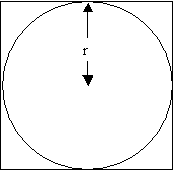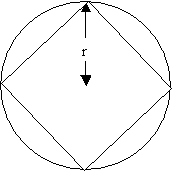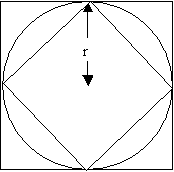#### You may also like### Contact

A circular plate rolls in contact with the sides of a rectangular tray. How much of its circumference comes into contact with the sides of the tray when it rolls around one circuit?### An Introduction to Irrational Numbers

Tim Rowland introduces irrational numbers

# Approximating Pi

##### Age 14 to 18 Challenge Level:

By inscribing a circle in a square and then a square in a circle find an approximation to pi.
By using a hexagon, can you improve on the approximation? How much better an approximation is it?

Archimedes used this idea first with a hexagon, then a dodecagon (12 sides) and so on up to a 96 sided polygon to calculate pi and was able to establish that $$3\frac{10}{71} < \pi < 3 \frac{1}{7}$$

What are the strengths and limitations of this method?